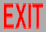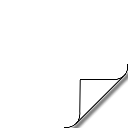# What is a particle?

## Classical physics

Classically, particles & waves are 2 different things.

### Classical mechanics

A classical particle is a point-like object. The type of particle is defined by properties that define how it interacts: mass (gravity) & charge (electromagnetism). At the subatomic level, there are generalizations of charge that describe interactions with short-range forces, but then quantum effects become important.

A free particle is described by its kinematic properties: energy, momentum, & angular momentum. Unlike the previous properties, energy & momentum take continuous values, but they are related by the mass: Nonrelativistically,

p⃗² = 2Km
while relativistically, in units c = 1 (length = time),
p⃗² = E² - m² = 2Km + K²
where E = m + K (rest energy + kinetic energy).* Of course, only relativistically can m = 0, since the nonrelativistic limit is K ≪ m. Classically, angular momentum can also take continuous values, but not quantum mechanically. These quantities are conserved because of how they relate to spacetime symmetries (through the action):
conservationsymmetry
energytime translation
momentumspace translation
angular momentum   rotation

*The relativistic quadratic equation has a 2nd solution, E ≈ - m - p⃗²/2m (antiparticle), but then K = E - m ≈ - 2m → - ∞ in the nonrelativistic limit.

### Classical field theory

Classically, interactions are described by fields, functions φ(x) of the spacetime coordinates (instead of the particle's x⃗(t), etc.). The analog of the energy-momentum relation is the "wave equation": nonrelativistically,
[(-i∇⃗)² - 2κ(i∂/∂t)] φ = 0
and relativistically,
[(-i∇⃗)² - (i∂/∂t)² + κ²] φ = 0
where κ is the analog of mass.

The analogs to the particle kinematic properties are

particlefield
mκ
E (or K)i∂/∂t
p⃗-i∇⃗
angular momentum   index
where "index" refers to whether the field is a vector φ⃗(x), scalar, tensor, spinor, etc. (Of course, the partial derivatives clearly relate to space & time translations.)

Energy & momentum can be expressed in terms of numbers, rather than (differential) operators, by Fourier transformation:

φ̃(k⃗,ω) ~ ∫dx⃗ dt exp(-ik⃗∙x⃗+iωt) φ(x⃗,t)
φ(x⃗,t) ~ ∫dk⃗ dω exp(ik⃗∙x⃗-iωt) φ̃(k⃗,ω)
so nonrelativistically
k⃗² = 2ωκ
& relativistically
k⃗² = ω² - κ²
If φ̃(k⃗,ω) is nonvanishing only for fixed values of k⃗ & ω (i.e., is proportional to Dirac δ-functions in them), φ(x⃗,t) is said to describe a "plane wave".

Except for scalar fields, the wave equations tend to be more complicated. In particular, the concept of a "gauge field" must be introduced. The result is that a free field must describe an irreducible representation of the Poincaré group (including space-time translations, rotations, & Lorentz boosts).

## Dimensional analysis

For this analogy, the engineering dimensions are not the same: Sticking with units c = 1 (length = time), for particles, m, E, & p⃗ have dimensions of mass, but for fields, "wave number" k, "frequency" ω, and κ all have dimensions of 1/length. Also, although the field index clearly relates to rotations, it has no relation to engineering dimensions, and is "quantized" in that there are only discrete types of index structure.

Fields also carry energy, momentum, & angular momentum, in the usual sense of how they appear in conservation laws & symmetry principles, but their form does not seem to resemble that of particles. In particular, they are defined as spatial integrals of local functions of the fields (with a few derivatives). There we find similar problems: Avoiding the introduction of ε₀ (& μ₀), the Coulomb force between electrons looks like

F ~ e²/r²
so (charge)² has dimensions mass × length, the dimensions of both angular momentum & "action". Specifically, it comes from a Coulomb potential in the action
S ~ e²∫dt 1/r
Alternatively, we could introduce a separate action for the photon, & write the total action as
S ~ ∫dt (mẋ² + ẋA) + (1/e²)∫dt d³x (∂A)²
where the "field" A then has dimensions of mass. (We have been sloppy with space & time indices on x, ∂, & A.)

On the other hand, the Yang-Mills action for a self-interacting field looks like

S ~ (1/g²)∫dt d³x (∂A + A²)²
So the field "A" has dimensions 1/length, contradicting what we found for coupling to the particle. Furthermore, from conservation laws the energy is
E ~ (1/g²)∫d³x (∂A + A²)²
(& similar for momentum; signs & indices work differently from S). From the usual dimensions for S & E, (charge)² now has dimensions 1/(mass × length), the inverse of what appears for particles. So if we add the actions of self-interacting fields to actions for particles with which they also interact, there will be a contradiction in dimensional analysis for not only fields, but also for coupling constants.

## Quantum physics

### Quantum mechanics

Quantum mechanics identifies particles & waves. This follows from the above comparisons once we fix the problem with engineering dimensions by introducing a constant "ℏ" with dimensions of action for the conversion. (Nowadays ℏ has been fixed to exactly 6.62607015×10⁻³⁴ J s/2π to define the kilogram, just as c has been fixed to exactly 299,792,458 m/s to define the meter.)

Note that, if we use consistent dimensions for energy & momentum, & thus the action, both for electron particle + Coulomb & for Yang-Mills, then the dimensionless quantities for the couplings are e²/ℏ for the former & g²ℏ for the latter. Thus the "classical" limit ℏ → 0 is strong coupling for the former & weak coupling for the latter. This relates to the fact that classical particles & classical fields are different limits of quantum theory. (Introducing interactions with particles @ the same time as self-interactions for fields makes the limit ℏ → 0 poorly defined.)

But the waves really appear in the Fourier expansion of a quantum mechanical wave function, not a field. This is much closer, but the full identification between particles & fields requires quantum field theory.

### Quantum field theory

Quantum field theory is a more powerful way to describe multiparticle states than quantum mechanics. In the same way, path integral (functional) quantization is a more powerful way to describe the quantum aspects of field theory than canonical quantization. The legacy method of canonical quantization of field theory uses an obscure construction involving harmonic oscillators to build multiparticle states as multiple excitations of a field "operator". But the functional expression is much simpler: Given a wave "functional" Ψ[φ], where the state Ψ depends on the field φ similarly to how φ depends on x⃗, the expansion into (a superposition of) various numbers of particles is simply a Taylor expansion of Ψ over φ:
Ψ[φ] = ψ₀ + ∫dx⃗ ψ₁(x⃗) φ(x⃗) + ½∫dx⃗₁ dx⃗₂ ψ₂(x⃗₁,x⃗₂) φ(x⃗₁) φ(x⃗₂) + ...
(For simplicity, we have described the nonrelativistic case. See "Fields" for the relativistic case.) Thus ψ₀ describes the "vacuum", ψ₁ is the wave function for a 1-particle state, ψ₂ for a 2-particle state, etc. The initial & final wave functionals are then inserted into the functional integral. Then one just evaluates the integral of a Gaussian (from the free action) times a polynomial (from the wave functionals & interaction terms in the action) to obtain Feynman diagrams.

In practice, one usually works with the Fourier transform version of the Taylor expansion in fields (i.e., just start in momentum space),

Ψ[φ] = ψ₀ + ∫dk⃗ ψ̃₁(k⃗) φ̃(k⃗) + ½∫dk⃗₁ dk⃗₂ ψ̃₂(k⃗₁,k⃗₂) φ̃(k⃗₁) φ̃(k⃗₂) + ...
(with 2π's distributed appropriately).

Not only is this construction conceptually simpler (working completely with functions instead of operators), but in the relativistic case it's manifestly Lorentz covariant @ each step. Canonical quantization, on the other hand, must take a detour into a Hamiltonian analysis & restore Lorentz invariance @ the end, a procedure that can become so complicated that no one ever uses it for the Standard Model. Yet many professors still torture their students by pretending canonical quantization of field theory is more "pedagogical", just like the way they teach freshmen physics as if it were still the 19th century.